• 回归分析是一种统计分析方法，用于研究多个统计量之间的关系，并利用关系进行预测。线性回归模型是最简单的回归分析模型，下面我们应用线性回归来预测票房。图8-5是计算机模拟生成的500个数据点，每个点表示一部电影...

回归分析是一种统计分析方法，用于研究多个统计量之间的关系，并利用关系进行预测。线性回归模型是最简单的回归分析模型，下面我们应用线性回归来预测票房。
图8-5是计算机模拟生成的500个数据点，每个点表示一部电影，横坐标是预告片搜索量，纵坐标是票房。图8-5称为散点图，是统计分析中最简单、最常用的图，用于对数据的规律做初步观察。观察图8-5可以发现，这些数据点大多分布在一条直线附近，这条直线代表了这些数据的分布规律，线性回归要做的就是根据散点图找到这条直线，这一过程也称为线性拟合。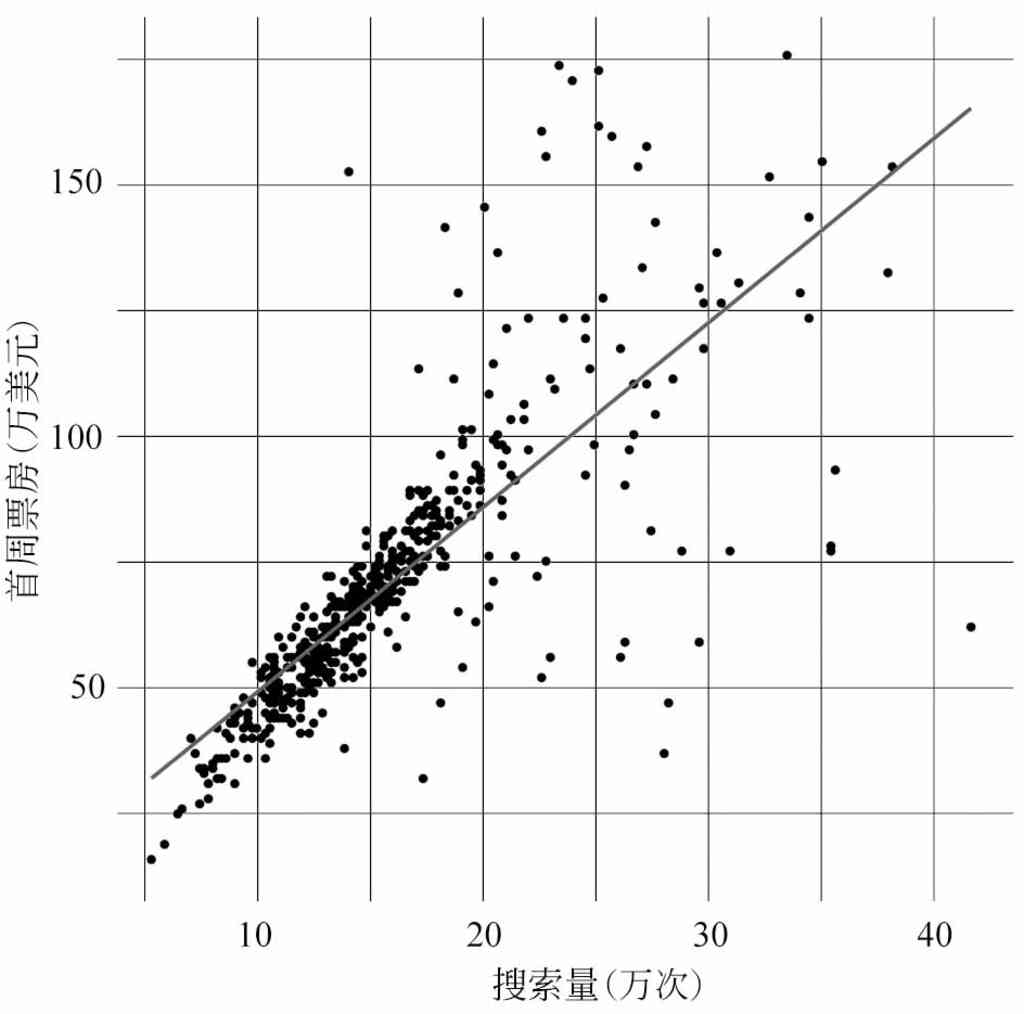图8-5　首周票房和预告片搜索量的散点图
设拟合直线的方程是y=ax+b，x表示预告片搜索量，y表示首周票房。线性回归的目标是找到最能体现数据特征的直线，也就是说，这条直线需要尽可能地“接近”所有数据。衡量多个点和一条直线之间的“接近程度”，最常用的指标是误差平方和。图8-6是误差平方和的一个示意图，基础数据包含4个点(图中的空心圆圈)，这四个点的X坐标分别对应拟合直线上的四个Y坐标，图中四条虚线的长度的平方和就是误差平方和，使误差平方和最小的那条直线就是最佳拟合直线，这种求解方法也称为最小二乘回归法。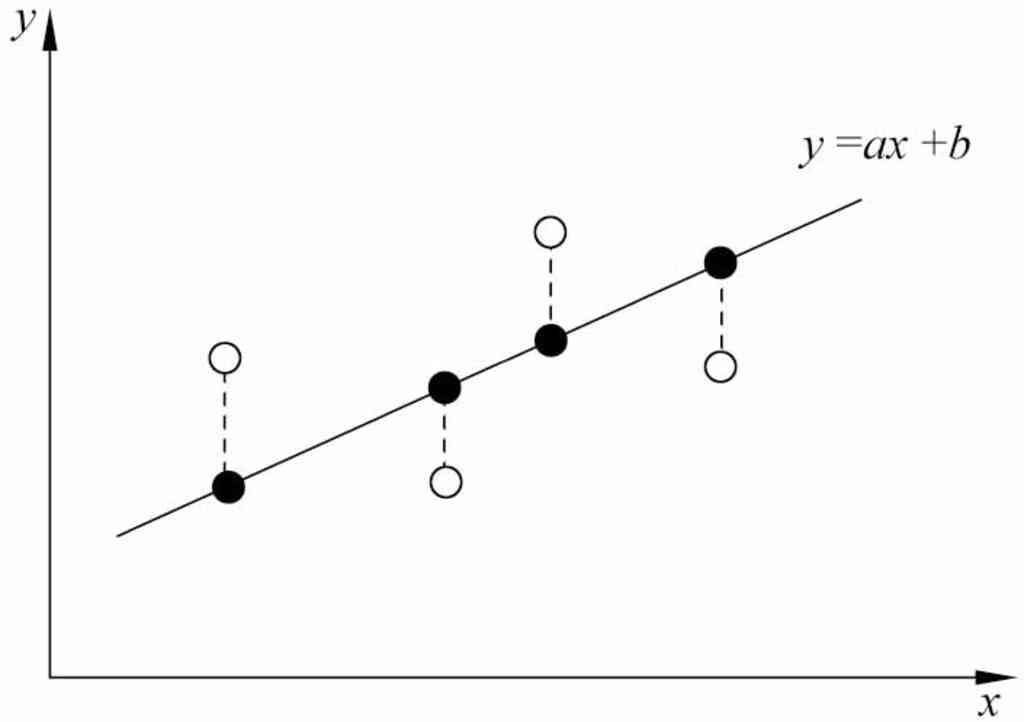图8-6　误差平方和示意图
当误差平方和达到最小值时，可以计算出a和b的值为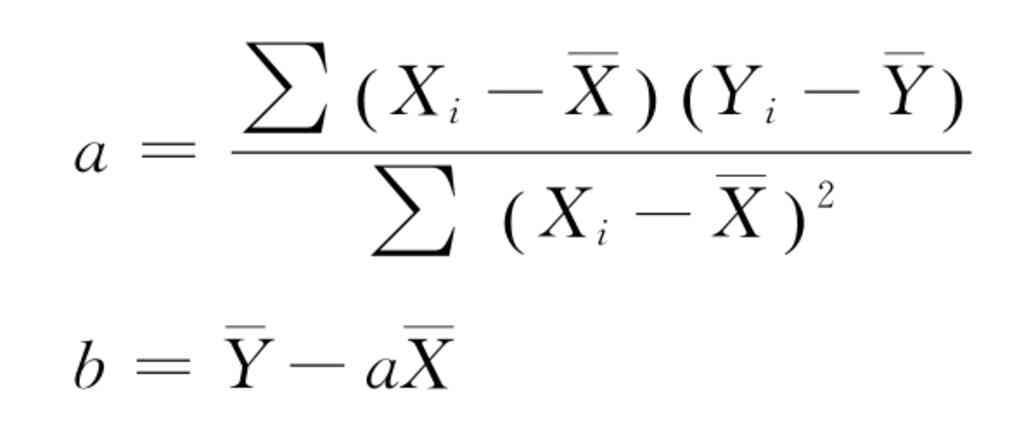至此便计算出了最佳拟合直线的表达式。
在处理线性回归问题时，我们可以把数据代入公式中进行计算，也可以使用统计软件，如Excel、R、SPSS等常用统计软件都有线性回归函数，我们只需要做少量的操作或编码就可以计算出线性回归的结果。
经计算，票房和搜索量的线性回归直线方程是：
y=3.5x+13.6
这条直线代表了票房和搜索量之间的关系，如图8-7所示。我们可以使用这条直线来预测票房，比如，某部即将上映的影片，预告片搜索量是12万次，即x=12，根据直线方程可以计算出y=55.6，因此我们预测这部影片的首周票房是55.6万美元。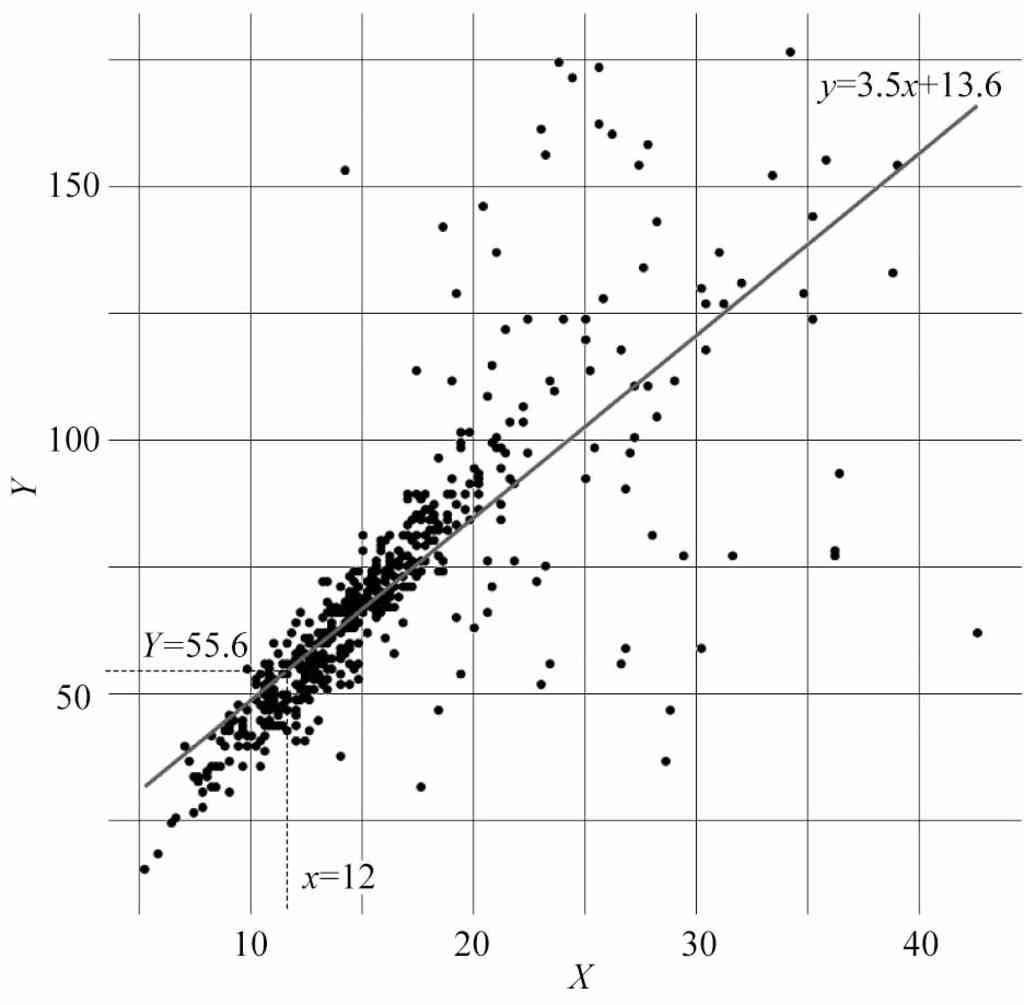图8-7　线性回归结果
除了直线方程，我们还可以计算另一个量化指标——相关系数。相关系数可以帮助我们判断两个变量的线性相关关系。此前，我们观察散点图，已经发现票房和搜索量之间近似存在线性相关关系，这只是感性判断，相关系数是对线性相关关系的理性判断。
相关系数r的计算公式为
r=a×Sx/Sy
式中，a是直线方程中的a，Sx表示X的标准差，Sy表示Y的标准差。如图8-8所示，r可以是-1～1的任意数值，其中最特别的三个数值是-1、1和0，含义如下：
r=-1表示y和x存在负相关关系，即a是负数；
r=1表示y和x存在正相关关系，即a是正数；
r=0表示y和x不存在任何线性相关关系，即a=0，不存在拟合直线。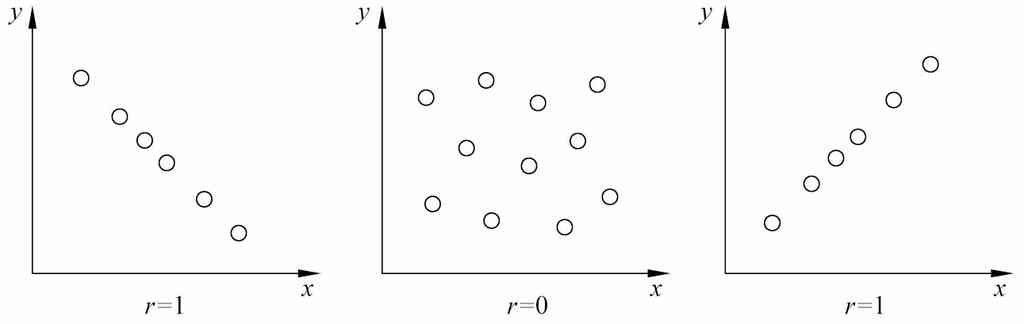图8-8　线性相关系数r的示意图
在实际问题中，r的值大多不会是-1、1或0，但我们可以借助它们的含义来判断线性相关关系。比如，当r=0.9时，我们认为r的值接近1，y和x存在近似的正相关关系；当r=-0.9时，我们认为r的值接近-1，y和x存在近似的负相关关系；当r=0.05时，我们认为r的值接近0，y和x几乎不存在线性相关关系。
至此，我们计算出了线性回归方程和线性相关系数。

展开全文• 数据可视化——R语言绘制散点相关图并自动添加相关系数和拟合方程加载所需的包并设置主题样式示例数据基本的散点相关图添加相关系数和显著性水平(P值)存在多个组别的散点相关图自动添加回归曲线的拟合方程拟合多项式...


数据可视化——R语言绘制散点相关图并自动添加相关系数和拟合方程
加载所需的包并设置主题样式示例数据基本的散点相关图添加相关系数和显著性水平(P值)存在多个组别的散点相关图自动添加回归曲线的拟合方程拟合多项式方程

概述：通常，我们需要对两个连续变量进行相关性分析，可采用散点图叠加拟合曲线（有时也可包含95%置信区间）来呈现相关性分析的结果。本文将介绍使用R语言来绘制散点相关图，并自动添加相关系数和显著性水平(P值)及拟合方程。
使用工具： R语言中的ggplot2包、ggpubr包和ggpmisc包
本文大部分内容来自一篇英文博客的翻译内容，博客原文链接：Plot Two Continuous Variables: Scatter Graph and Alternatives，但笔者也对一些参数进行了修改，使得图形更为美观。
加载所需的包并设置主题样式
library(ggplot2)
library(ggpubr)
library(ggpmisc)
theme_set(ggpubr::theme_pubr()+
theme(legend.position = "top"))

示例数据
示例数据集：mtcars。变量 cyl 作为分组变量。
# Load data
data("mtcars")
df <- mtcars
# Convert cyl as a grouping variable
df$cyl <- as.factor(df$cyl)
# Inspect the data
head(df[, c("wt", "mpg", "cyl", "qsec")], 4)

示例数据如下：
##                  wt  mpg cyl qsec
## Mazda RX4      2.62 21.0   6 16.5
## Mazda RX4 Wag  2.88 21.0   6 17.0
## Datsun 710     2.32 22.8   4 18.6
## Hornet 4 Drive 3.21 21.4   6 19.4

基本的散点相关图
关键函数：
geom_point()：用于创建散点图。关键参数：color，size和shape，用于设置散点的边缘颜色，大小和形状。  geom_smooth()：用于添加回归曲线和曲线拟合的置信区间。关键参数：
color，size和linetype：指定回归曲线的颜色、粗细和线型。fill：指定置信区间的填充颜色。method：用于指定曲线拟合的方法。
b <- ggplot(df, aes(x = wt, y = mpg))
# Scatter plot with regression line
b + geom_point()+
geom_smooth(method = "lm", color = "black", fill = "lightgray")

# Add a loess smoothed fit curve
b + geom_point()+
geom_smooth(method = "loess", color = "black", fill = "lightgray")注意：如果不需要显示曲线拟合的置信区间(即上图中的阴影区域)，可以设置 geom_smooth() 参数se = FALSE。
散点图点的形状可由 geom_point() 中参数 shape 指定，例如：
b + geom_point(shape = 17)+
geom_smooth(method = "lm", color = "black", fill = "lightgray")键入如下命令，可以查看R中点的形状及对应的索引：
ggpubr::show_point_shapes()添加相关系数和显著性水平(P值)
使用 ggscatter() 也可以轻松绘制散点图，并可以使用 stat_cor() 自动添加相关系数和显著性水平(P值)。
# Add regression line and confidence interval
ggscatter(df, x = "wt", y = "mpg",
add = "reg.line", conf.int = TRUE,

)+
stat_cor(method = "pearson",
label.x = 3, label.y = 30)存在多个组别的散点相关图
依据组别改变点的颜色和形状  使用函数 geom_rug() 在X轴和Y轴上添加轴须线(marginal rug)
# Change color and shape by groups (cyl)
b + geom_point(aes(color = cyl, shape = cyl))+
geom_smooth(aes(color = cyl, fill = cyl), method = "lm") +
geom_rug(aes(color =cyl)) +
scale_color_manual(values = c("#00AFBB", "#E7B800", "#FC4E07"))+
scale_fill_manual(values = c("#00AFBB", "#E7B800", "#FC4E07"))
# Remove confidence region (se = FALSE)
# Extend the regression lines: fullrange = TRUE
b + geom_point(aes(color = cyl, shape = cyl)) +
geom_rug(aes(color =cyl)) +
geom_smooth(aes(color = cyl), method = lm,
se = FALSE, fullrange = TRUE)+
scale_color_manual(values = c("#00AFBB", "#E7B800", "#FC4E07"))+
ggpubr::stat_cor(aes(color = cyl), label.x = 3)使用 facet_wrap() 将各个分组展示于不同面板：
b + geom_point(aes(color = cyl, shape = cyl))+
geom_smooth(aes(color = cyl, fill = cyl),
method = "lm", fullrange = TRUE) +
facet_wrap(~cyl) +
scale_color_manual(values = c("#00AFBB", "#E7B800", "#FC4E07"))+
scale_fill_manual(values = c("#00AFBB", "#E7B800", "#FC4E07")) +
theme_bw()自动添加回归曲线的拟合方程
使用 stat_poly_eq() 可以将回归曲线的拟合方程添加到图形中。值得注意的是，stat_poly_eq() 还可以用于添加拟合方程的决定系数(R2)、P值、AIC值和BIC值等，可通过设定label 实现。
b <- ggplot(df, aes(x = wt, y = mpg))

formula <- y ~ x

b + geom_point(shape = 17)+
geom_smooth(method = "lm", color = "black", fill = "lightgray") +
stat_cor(method = "pearson",label.x = 3, label.y = 30) +
stat_poly_eq(
aes(label = ..eq.label..),
formula = formula,parse = TRUE, geom = "text",label.x = 3,label.y = 28, hjust = 0)注意： 显示相关系数与方程式的两个文本的位置需要根据实际的数据分布作调整，尽量显示在没有点分布的位置处。
为了将显示相关系数与方程式的两个文本对齐，上图为左对齐，需设置 stat_cor() 和 stat_poly_eq() 中label.x的值相同（横轴位置相同）， 设置label.y来控制两文本的间隔。同时，需要设置 stat_poly_eq() 中geom = “text”。此外，在指定的 (label.x, label.y) 位置处显示文本，stat_cor() 默认为左对齐，而 stat_poly_eq() 默认为居中对齐，为了保持一致，需将 stat_poly_eq() 也设置为左对齐，即hjust = 0。(注：hjust=0表示左对齐，hjust=1表示右对齐，hjust=0.5表示居中对齐)
上图是通过指定显示文本的绝对位置来实现，即 label.x 和 label.y 的取值范围与X轴和Y轴的取值对应。
也可以通过以下方式来实现：其中通过 label.x.npc 和 label.y.npc 来指定显示文本的位置，但 label.x.npc 和 label.y.npc的取值范围为0~1，如0.5表示居中。
b <- ggplot(df, aes(x = wt, y = mpg))

formula <- y ~ x

b + geom_point(shape = 17)+
geom_smooth(method = "lm", color = "black", fill = "lightgray") +
stat_cor(method = "pearson",label.x.npc = 0.5, label.y.npc = 0.9) +
stat_poly_eq(
aes(label = ..eq.label..),
formula = formula,parse = TRUE,label.x.npc = 0.5,label.y.npc = 0.8, hjust = 0)

拟合多项式方程
创建样本数据
set.seed(4321)
x <- 1:100
y <- (x + x^2 + x^3) + rnorm(length(x), mean = 0, sd = mean(x^3) / 4)
my.data <- data.frame(x, y, group = c("A", "B"),
y2 = y * c(0.5,2), block = c("a", "a", "b", "b"))

采用多项式回归拟合并添加拟合方程
# Polynomial regression. Sow equation and adjusted R2
formula <- y ~ poly(x, 3, raw = TRUE)
p <- ggplot(my.data, aes(x, y2, color = group)) +
geom_point() +
geom_smooth(aes(fill = group), method = "lm", formula = formula) +
stat_poly_eq(
aes(label =  paste(..eq.label.., ..adj.rr.label.., sep = "~~~~")),
formula = formula, parse = TRUE
)+
scale_fill_manual(values = c("#00AFBB", "#E7B800"))+
scale_color_manual(values = c("#00AFBB", "#E7B800"))
p注意：可以在 label 中添加 ..AIC.label.. 和 ..BIC.label.. ，
将会显示拟合方程的AIC值和BIC值。

stat_poly_eq()中的 label.x 和 label.y 可用于调整标签显示的位置。

想要查看更多的示例，请键入该命令进行查看：
browseVignettes("ggpmisc")
将打开如下网页：http://127.0.0.1:18537/session/Rvig.2970595b7d23.html

References
原文链接：Plot Two Continuous Variables: Scatter Graph and Alternatives
展开全文数据可视化
• ) p ggsave("tmp1.pdf",width = 8,height = 8,units = "cm") 如果想添加拟合的方程，可以使用ggpmisc包的stat_poly_eq()函数，该函数可以拟合多项式方程并生成多种标签，比如p值、决定系数R方，这些由函数计算出来的...

属于比较常用的技巧。下面我用ggplot2内置数据集进行演示：

先来看一下，仅有散点和拟合线的图
df=diamonds[sample(1:dim(diamonds),40),]
df$price=df$price / 10000
p=df%>%ggplot(aes(carat,price))+
geom_point(size=4,alpha=0.3,color="#6baed6")+
geom_smooth(method = "lm", formula = y~x, color = "#756bb1", fill = "#cbc9e2")+ #颜色选自https://colorbrewer2.org/
theme_bw()+
theme(
panel.grid.major = element_blank(),panel.grid.minor = element_blank()
)
p
ggsave("tmp1.pdf",width = 8,height = 8,units = "cm")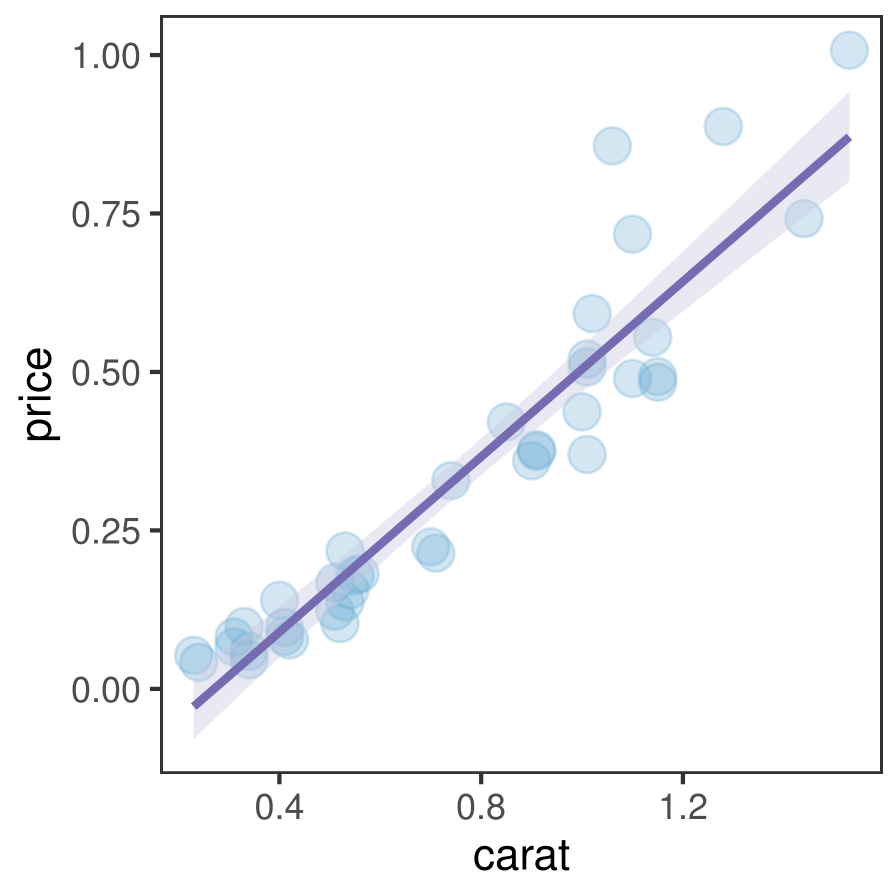如果想添加拟合的方程，可以使用ggpmisc包的stat_poly_eq()函数，该函数可以拟合多项式方程并生成多种标签，比如p值、决定系数R方，这些由函数计算出来的量通常名称前后有两个点
..eq.label..
..p.value.label..
对应的代码只有一行，其余代码和上面一样：
library(ggpmisc)
#这一行paste()中的内容是一个类似Latex的表达，“~”表示空格，parse=T表示将这个语句翻译成可读形式；size=2.5表示字体大小
ggsave("tmp2.pdf",width = 8,height = 8,units = "cm")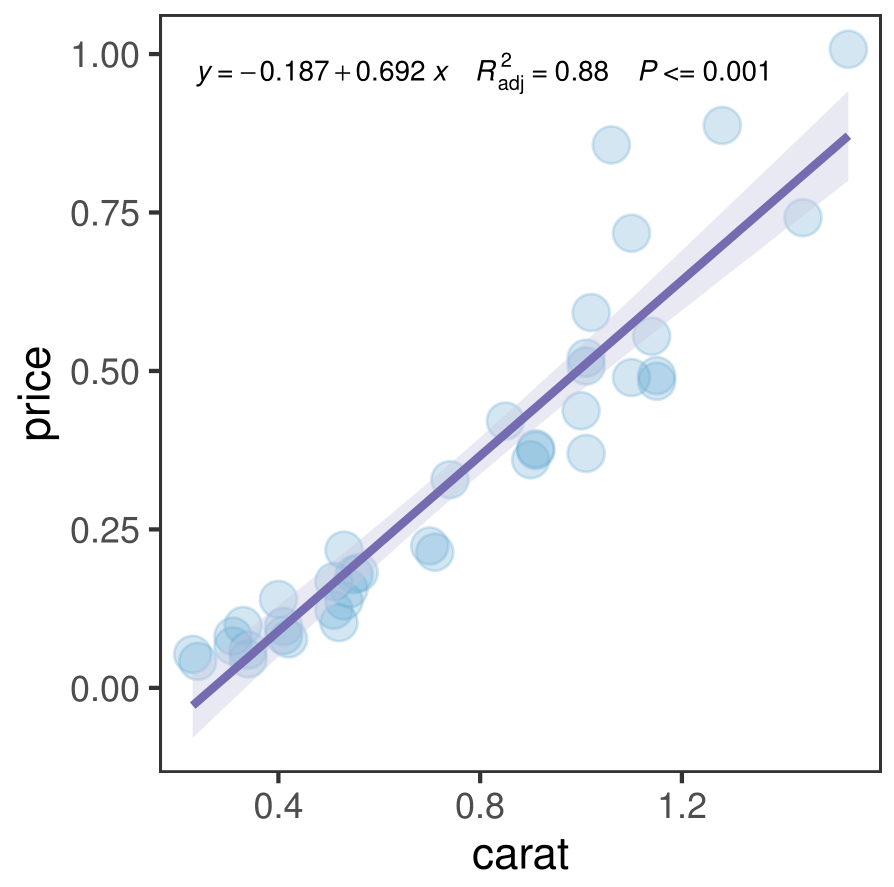如果需要添加相关系数，可以按照相同的思路，先求出对应的值，再加到stat_poly_eq()的label参数里
cor=round(cor(df$carat,df$price),2)
p+stat_poly_eq(aes(label=paste("italic(r)~=~",cor,sep = "")),formula = y~x,parse=T,size=4)
ggsave("tmp3.pdf",width = 8,height = 8,units = "cm")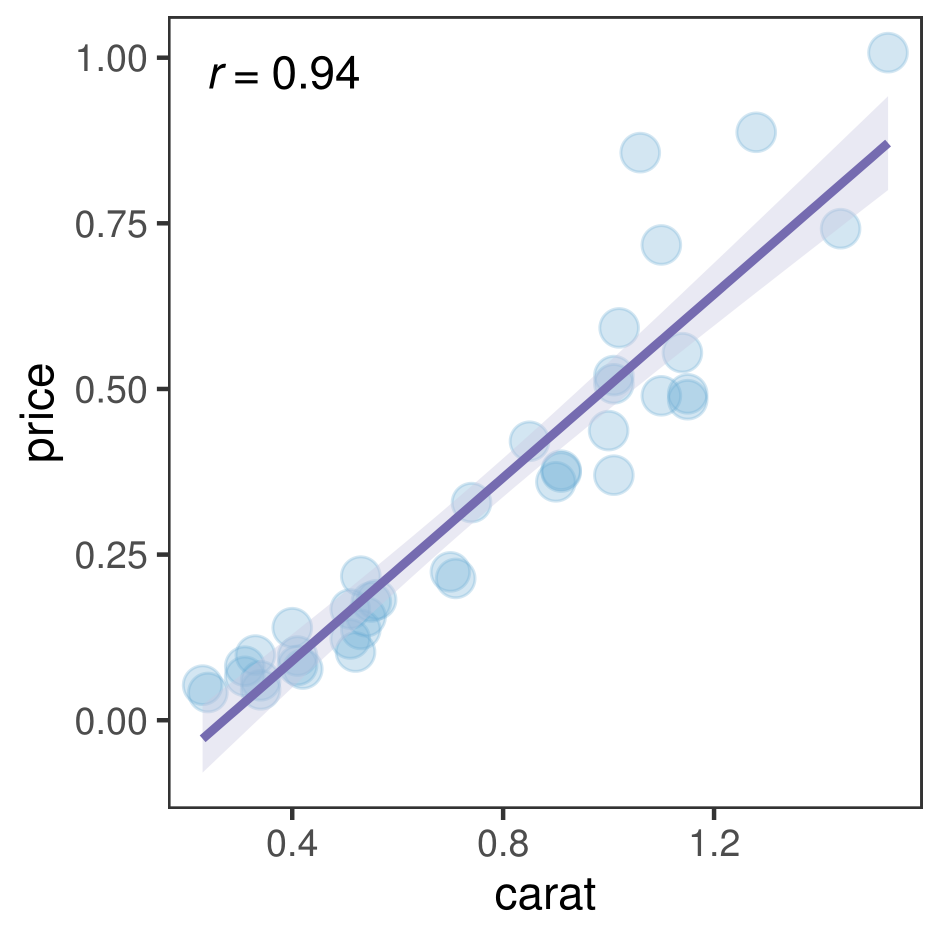另外也可以使用ggpubr包的stat_cor()函数，添加相关系数以及p值
library(ggpubr)
p+stat_cor()
ggsave("tmp4.pdf",width = 8,height = 8,units = "cm")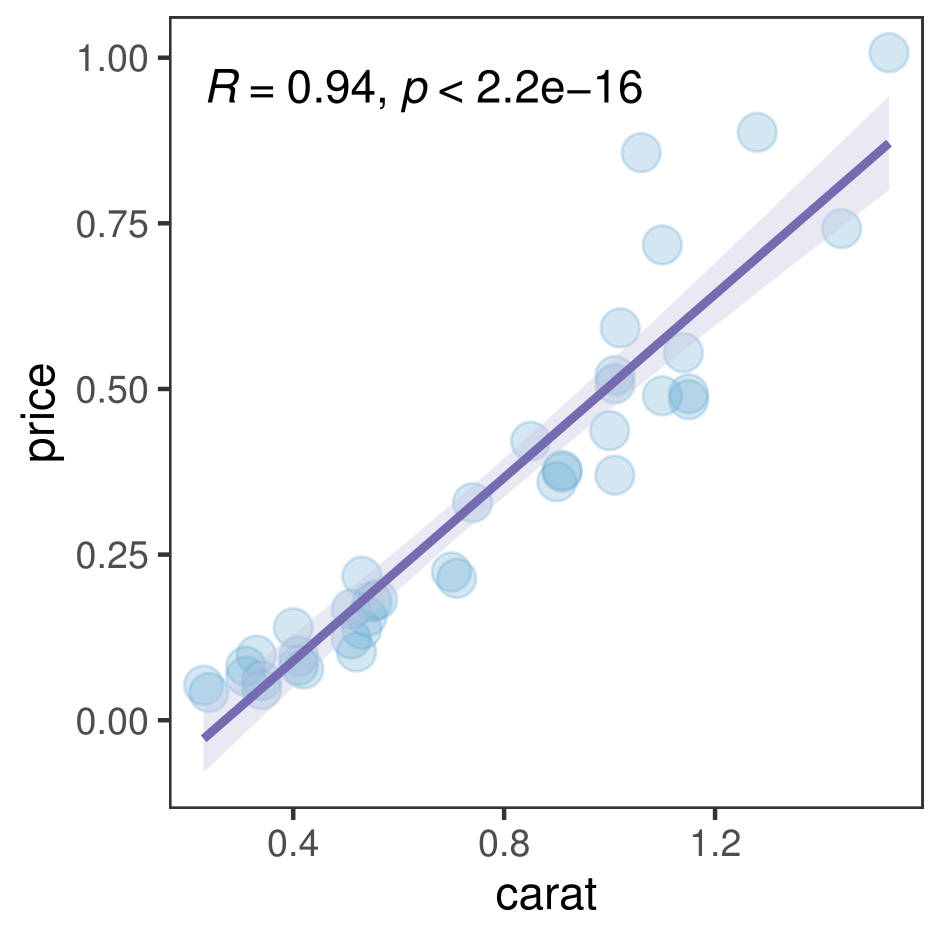将stat_poly_eq()和stat_cor()结合起来也是可以的，当然两个文本的位置需要调整一下
p+stat_poly_eq(aes(label=..eq.label..),formula = y~x,parse=T,size=4)+
stat_cor(label.y=0.9,size=4)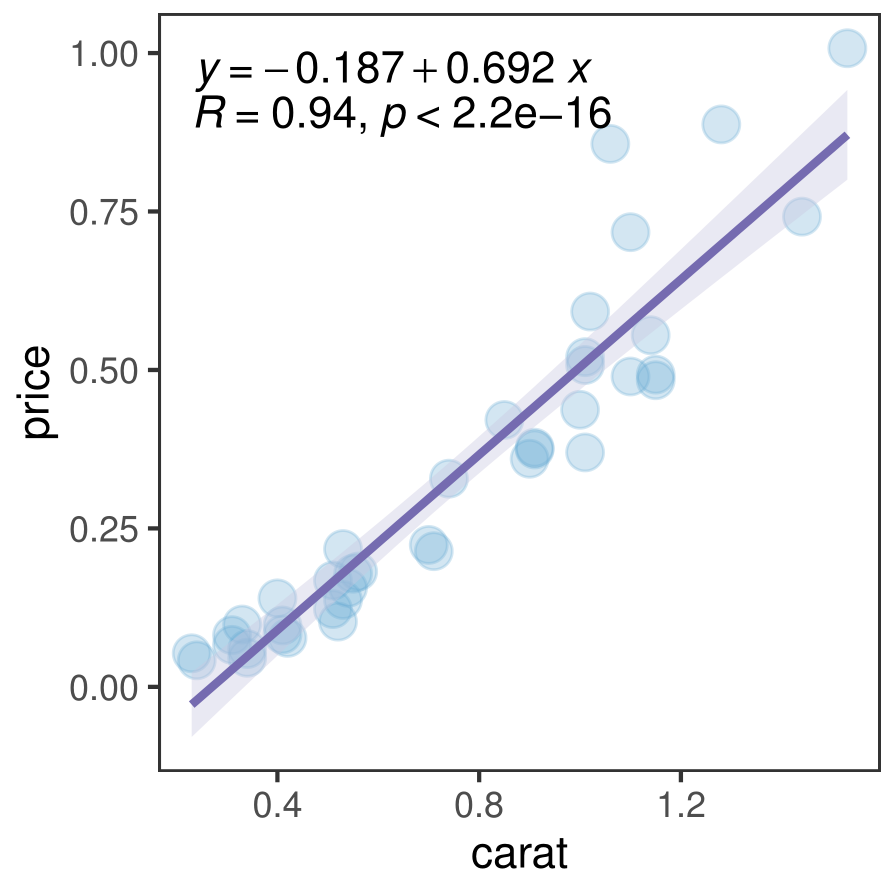因水平有限，有错误的地方，欢迎批评指正！

展开全文• 1 皮尔逊相关系数（Pearson Correlation Coefficient） 皮尔逊相关系数广泛用于度量两个变量之间的相关程度，其值介于-1与1之间。 两个变量之间的皮尔逊相关系数定义为两个变量之间的协方差和标准差的商： 上式...
1 皮尔逊相关系数（Pearson Correlation Coefficient）
皮尔逊相关系数广泛用于度量两个变量之间的相关程度，其值介于-1与1之间。
两个变量之间的皮尔逊相关系数定义为两个变量之间的协方差和标准差的商：上式定义了总体相关系数，常用希腊小写字母作为代表符号。估算样本的协方差和标准差，可得到皮尔逊相关系数，常用英文小写字母 
展开全文• 每次比赛都需要查一下，这次直接总结到自己的博客中。 以这个为例子： 2.线性方程相关计算 x=[1,2,3,4,5]';%参数矩阵 ...[b,bint,r,rint,stats]=regress(Y,X) ...%b为方程相关系数 %r表示...
• 文章目录 十一、回归方程与回归系数的显著性检验 1.平方和分解 2.回归方程的假设检验 3.中心化的等价形式 4.回归系数的假设检验 5.回归方程的预报精度 回顾总结 十一、回归方程与回归系数的显著性检验 回归方程的...多元统计分析 假设检验
• 1.一元线性回归方程 2.多元线性回归方程
• 回归中的相关系数以及R平方值和Python应用举例 1. 皮尔逊相关系数 (Pearson Correlation Coefficient): 1.1 衡量两个值线性相关强度的量 1.2 取值范围 [-1, 1]: 正向相关: >0, 负向相关： 2. R平方值:...
• 一、内在关系 直线回归相关的性质或方向（正或负）...（1）相关系数是标准化的回归系数  回归系数b是有单位的，但若对b作消去单位的标准化处理，即对b中x,y的离均差以各自的标准差 和 为单位：      ...
• 相关系数(r)可以衡量这种相关关系。r的取值范围是[-1,1]，r=1表示完全正相关r=-1表示完全负相关r=0表示完全不相关。为什么要对相关系数进行显著性检验？1）实际上完全没有关系的变量，在利用样本数据进行计算时...
• regress（）函数主要用于...bint是回归系数的区间估计，r是残差，rint是置信区间，stats是用于检验回归模型的统计量，有三个数值：相关系数r^2，F值，与F对应的概率P，alpha是显著性水平(缺省的时候为0.05)。相关系数r
• 检查回归方程的有效性 回归分析之前 1 对y进行正态分布的检验,如果y不服从正态分布，则需要进行变换。 2 通过相关性分析，判断x与y的相关性，如果不相关，则不需要纳入此x到方程中。 3 通过相关性...残差分析 F检验 t检验 多重共线性
• 基于jupyter notebook的python编程-----通过原理，求解分析多元线性回归方程的的待定系数a和判定系数R2目录一、二元线性回归的推导原理1、因变量百度y关于自标量nxxx21,的多元回归方程如下：2、离散化数据（m组...python 机器学习 csv excel 人工智能
• 分析线性回归方程的的待定系数a和判定系数R2的分解步骤目录一、运行jupyter notebook，搭建python环境1、打开Windows终端命令行，输入==jupyter notebook==，打开我们的jupyter工具，如下所示：2、在jupyter的web...python 机器学习 人工智能 算法 深度学习
• %%1、bint表示回归系数区间估计 %2、r表示残差 %3、rint代表置信区间 ...% r^2越接近于1，回归方程越显著 %alpha表示显著水平 %% x=[143 144 145 147 148 150 153 154 155 156 157 158 159 160 1...
• 线性回归是机器学习中的基础算法之一，属于监督学习中的回归问题，算法的关键在于如何最小化代价函数，通常使用梯度下降或者正规方程（最小二乘法），在这里对算法原理不过多赘述，建议看吴恩达发布在斯坦福大学上的...
• 背景计算线性回归a、b值后，希望计算r-squared，对比是否为最佳拟合Excel拟合数据源拟合结果Python拟合import mathimport numpy as npfrom scipy import statstestX = [174.5,171.2,172.9,161.6,123.6,112.1,107.1,...
• 目录一、基础知识二、回归系数相关系数1.定义2.二者的联系3.二者的区别 假设有两个随机变量(x,y)(x,y)(x,y)，其NNN个样本组合为（x1,x2,…,xN）（x_1,x_2,\dots,x_N）（x1​,x2​,…,xN​）和(y1,y2,…,yN)(y_1,y_...
• 一元二次回归方程计算方法，使用最小二乘法计算。。回归分析 一元二次回归方程计算方法，使用最小二乘法计算。。回归分析
• 线性回归方程式 线性回归遵循如下方程式 y=m*x+b。 m = SLOPE(Data_Y;Data_X) b = INTERCEPT(Data_Y ;Data_X) 计算决定系数通过 r² = RSQ(Data_Y;Data_X) 除了 m, b 和 r² 之外，数组函数 ...
•  （3）利用最小二乘法求出估计的回归方程，并解释回归系数的实际意义。 （4）计算判定系数，并解释其意义。 import matplotlib.pyplot as plt import numpy as np x=np.array([22460,11226,34547,4851,5444,2662,...
• c# 拟合曲线 求相关系数R 线性拟合y=kx+b；多项式拟合
• a、多重判定系数反应反映了因变量 y 的变差中估计的回归方程所解释的比例 b、    的平方根称为多重相关系数，也称为复相关系数，它度量了因变量同 k 个自变量的相关程度 ...
• 1.确定回归系数的点估计值： b = regress(Y,X) 2.求回归系数的点估计和区间估计、并检验回归模型： ...stats ：用于检验回归模型的统计量，有三个数值：相关系数r的平方、F值、与F对应的概率p a...数学建模 matlab
• 如果你已经有了一堆数据，有了因变量和自变量，利用统计软件求出回归方程是非常简单的事情，这些软件虽然不懂得如何分辨你的数据应该用什么方法，但是一旦你确定了方法，他们的计算能力还是非常强大的，可以快速地给...数据分析 数据挖掘
• 分:秒:微秒): 00:00:00:241优化算法: 麦夸特法(Levenberg-Marquardt) + 通用全局优化法计算结束原因: 达到收敛判断标准均方差(RMSE): 0.0274286872091944残差平方和(SSE): 0.00752332882019822相关系数(R):...
• #define NUM 14 int main() {  int n = 14;... //回归分析  int temperature[] = { 29,28,34,31,25,29,32,31,24,33,25,31,26,30 };  int sales[] = { 77,62,93,84,59,64,80,75,58,91,51,73,65,84 };
• 欢迎关注”生信修炼手册”!回归分析是一种广泛使用的统计工具，利用已有的实验数据...回归分析有两个经典的用法，第一个就是建模预测，通过构建的回归方程来对新的数据集进行预测，第二个就是用于定量描述变量间的相......